# Excel Excellence (Microsoft Excel training that "sticks"): Formulas

Oct. 12, 2014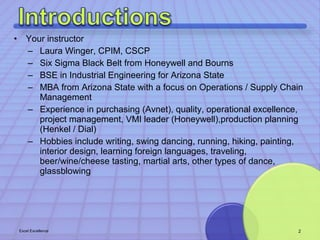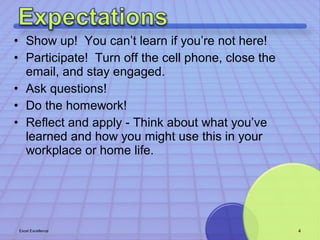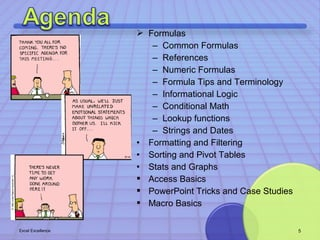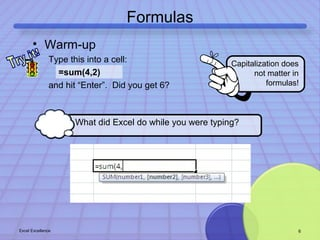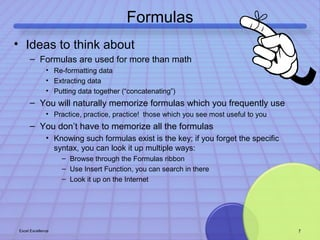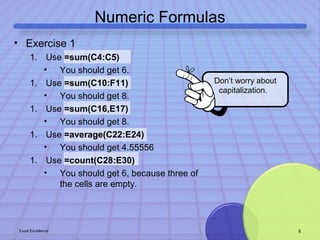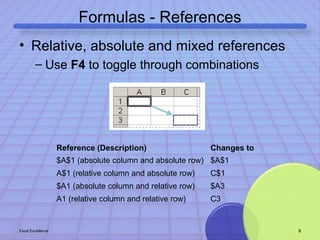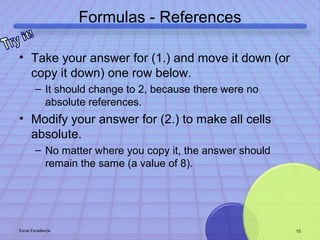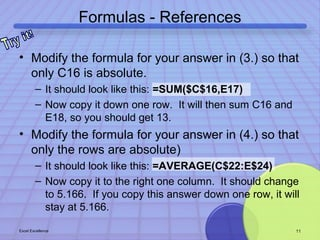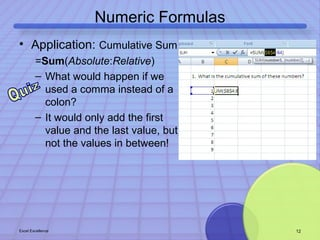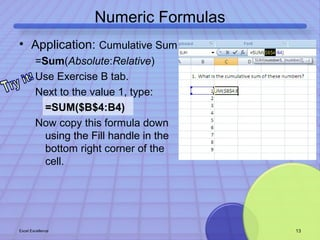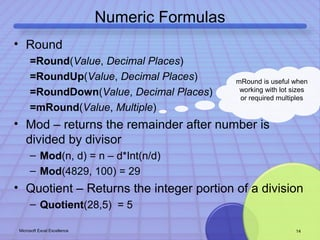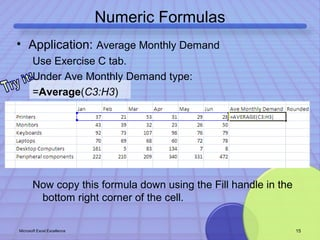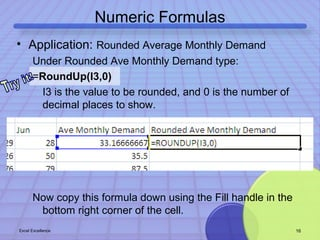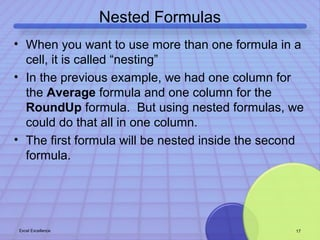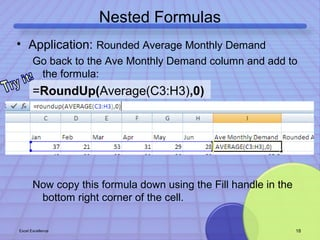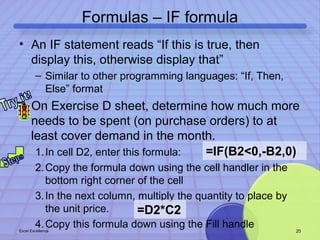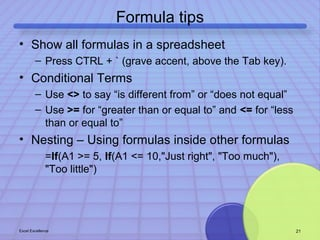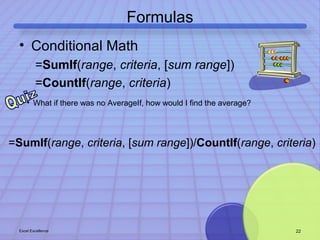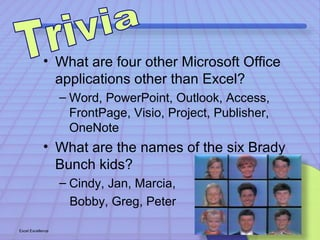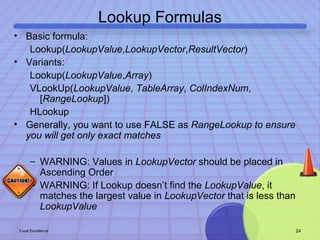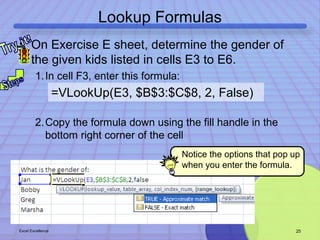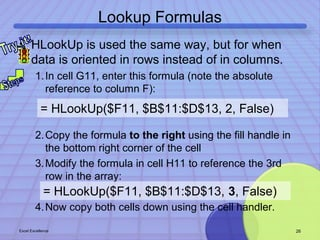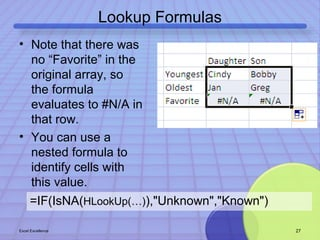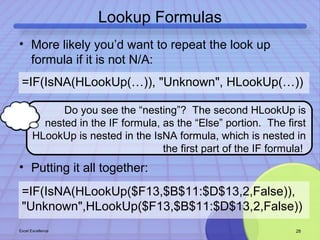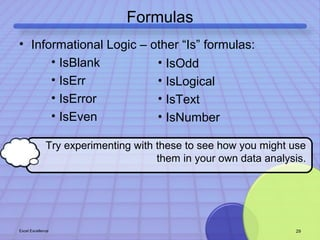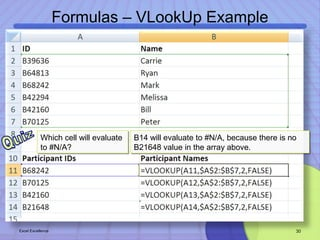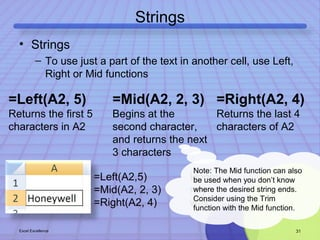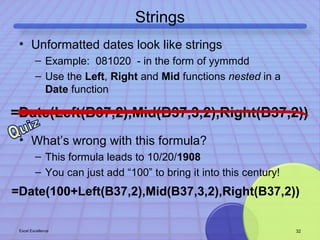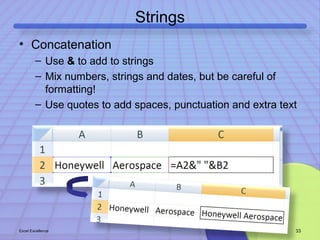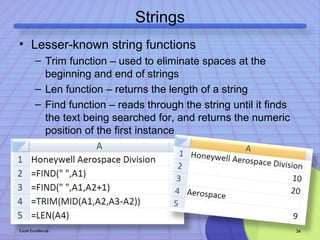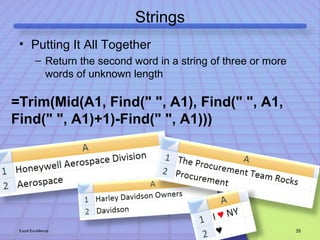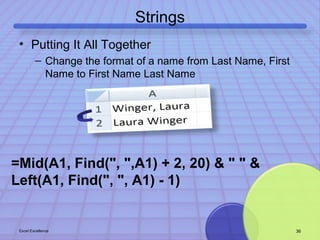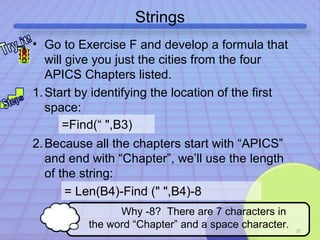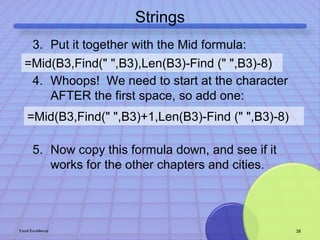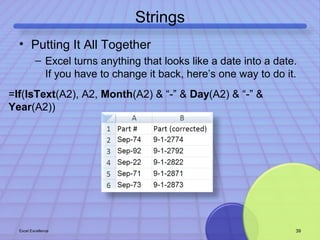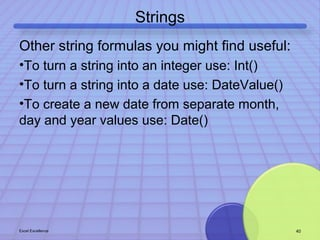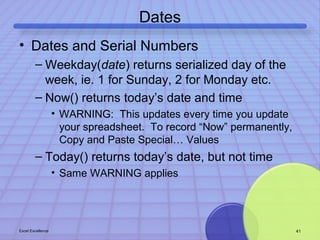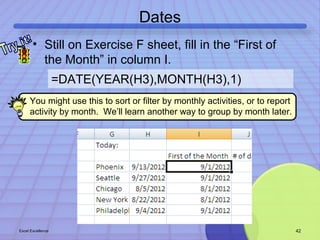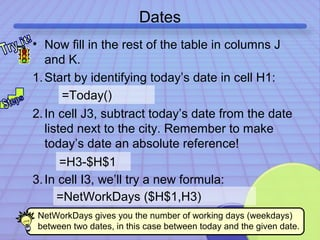1 of 44

### Excel Excellence (Microsoft Excel training that "sticks"): Formulas

• 1. Excel training that “sticks” by Laura Winger
• 2. • Your instructor – Laura Winger, CPIM, CSCP – Six Sigma Black Belt from Honeywell and Bourns – BSE in Industrial Engineering for Arizona State – MBA from Arizona State with a focus on Operations / Supply Chain Management – Experience in purchasing (Avnet), quality, operational excellence, project management, VMI leader (Honeywell),production planning (Henkel / Dial) – Hobbies include writing, swing dancing, running, hiking, painting, interior design, learning foreign languages, traveling, beer/wine/cheese tasting, martial arts, other types of dance, glassblowing Excel Excellence 2
• 3. “It is when the tools of production are transparent, that we are inspired to create.” - Chris Anderson, The Long Tail
• 4. • Show up! You can’t learn if you’re not here! • Participate! Turn off the cell phone, close the email, and stay engaged. • Ask questions! • Do the homework! • Reflect and apply - Think about what you’ve learned and how you might use this in your workplace or home life. Excel Excellence 4
• 5.  Formulas – Common Formulas – References – Numeric Formulas – Formula Tips and Terminology – Informational Logic – Conditional Math – Lookup functions – Strings and Dates • Formatting and Filtering • Sorting and Pivot Tables • Stats and Graphs  Access Basics  PowerPoint Tricks and Case Studies  Macro Basics Excel Excellence 5
• 6. Formulas Capitalization does • Warm-up Type this into a cell: =sum(4,2) and hit “Enter”. Did you get 6? What did Excel do while you were typing? not matter in formulas! Excel Excellence 6
• 7. Formulas • Ideas to think about – Formulas are used for more than math • Re-formatting data • Extracting data • Putting data together (“concatenating”) – You will naturally memorize formulas which you frequently use • Practice, practice, practice! those which you see most useful to you – You don’t have to memorize all the formulas • Knowing such formulas exist is the key; if you forget the specific syntax, you can look it up multiple ways: – Browse through the Formulas ribbon – Use Insert Function, you can search in there – Look it up on the Internet Excel Excellence 7
• 8. Numeric Formulas Don’t worry about capitalization. • Exercise 1 1. Use =sum(C4:C5) • You should get 6. 1. Use =sum(C10:F11) • You should get 8. 1. Use =sum(C16,E17) • You should get 8. 1. Use =average(C22:E24) • You should get 4.55556 1. Use =count(C28:E30) • You should get 6, because three of the cells are empty. Excel Excellence 8
• 9. Formulas - References • Relative, absolute and mixed references – Use F4 to toggle through combinations Reference (Description) Changes to \$A\$1 (absolute column and absolute row) \$A\$1 A\$1 (relative column and absolute row) C\$1 \$A1 (absolute column and relative row) \$A3 A1 (relative column and relative row) C3 Excel Excellence 9
• 10. Formulas - References • Take your answer for (1.) and move it down (or copy it down) one row below. – It should change to 2, because there were no absolute references. • Modify your answer for (2.) to make all cells absolute. – No matter where you copy it, the answer should remain the same (a value of 8). Excel Excellence 10
• 11. Formulas - References • Modify the formula for your answer in (3.) so that only C16 is absolute. – It should look like this: =SUM(\$C\$16,E17) – Now copy it down one row. It will then sum C16 and E18, so you should get 13. • Modify the formula for your answer in (4.) so that only the rows are absolute) – It should look like this: =AVERAGE(C\$22:E\$24) – Now copy it to the right one column. It should change to 5.166. If you copy this answer down one row, it will stay at 5.166. Excel Excellence 11
• 12. Numeric Formulas • Application: Cumulative Sum =Sum(Absolute:Relative) – What would happen if we used a comma instead of a colon? – It would only add the first value and the last value, but not the values in between! Excel Excellence 12
• 13. Numeric Formulas • Application: Cumulative Sum =Sum(Absolute:Relative) Use Exercise B tab. Next to the value 1, type: =SUM(\$B\$4:B4) Now copy this formula down using the Fill handle in the bottom right corner of the cell. Excel Excellence 13
• 14. Numeric Formulas • Round =Round(Value, Decimal Places) =RoundUp(Value, Decimal Places) =RoundDown(Value, Decimal Places) =mRound(Value, Multiple) • Mod – returns the remainder after number is divided by divisor mRound is useful when working with lot sizes or required multiples – Mod(n, d) = n – d*Int(n/d) – Mod(4829, 100) = 29 • Quotient – Returns the integer portion of a division – Quotient(28,5) = 5 Microsoft Excel Excellence 14
• 15. Numeric Formulas • Application: Average Monthly Demand Use Exercise C tab. Under Ave Monthly Demand type: =Average(C3:H3) Now copy this formula down using the Fill handle in the bottom right corner of the cell. Microsoft Excel Excellence 15
• 16. Numeric Formulas • Application: Rounded Average Monthly Demand Under Rounded Ave Monthly Demand type: =RoundUp(I3,0) I3 is the value to be rounded, and 0 is the number of decimal places to show. Now copy this formula down using the Fill handle in the bottom right corner of the cell. Excel Excellence 16
• 17. Nested Formulas • When you want to use more than one formula in a cell, it is called “nesting” • In the previous example, we had one column for the Average formula and one column for the RoundUp formula. But using nested formulas, we could do that all in one column. • The first formula will be nested inside the second formula. Excel Excellence 17
• 18. Nested Formulas • Application: Rounded Average Monthly Demand Go back to the Ave Monthly Demand column and add to the formula: =RoundUp(Average(C3:H3),0) Now copy this formula down using the Fill handle in the bottom right corner of the cell. Excel Excellence 18
• 19. Break Time oWhat did you learn? oWhere will you use it? oDo you have any questions?
• 20. Formulas – IF formula • An IF statement reads “If this is true, then display this, otherwise display that” – Similar to other programming languages: “If, Then, Else” format • On Exercise D sheet, determine how much more needs to be spent (on purchase orders) to at least cover demand in the month. =IF(B2<0,-B2,0) 1.In cell D2, enter this formula: 2.Copy the formula down using the cell handler in the bottom right corner of the cell 3.In the next column, multiply the quantity to place by the unit price. =D2*C2 4.Copy this formula down using the Fill handle Excel Excellence 20
• 21. Formula tips • Show all formulas in a spreadsheet – Press CTRL + ` (grave accent, above the Tab key). • Conditional Terms – Use <> to say “is different from” or “does not equal” – Use >= for “greater than or equal to” and <= for “less than or equal to” • Nesting – Using formulas inside other formulas =If(A1 >= 5, If(A1 <= 10,"Just right", "Too much"), "Too little") Excel Excellence 21
• 22. Formulas • Conditional Math =SumIf(range, criteria, [sum range]) =CountIf(range, criteria) • What if there was no AverageIf, how would I find the average? =SumIf(range, criteria, [sum range])/CountIf(range, criteria) Excel Excellence 22
• 23. • What are four other Microsoft Office applications other than Excel? – Word, PowerPoint, Outlook, Access, FrontPage, Visio, Project, Publisher, OneNote • What are the names of the six Brady Bunch kids? – Cindy, Jan, Marcia, Bobby, Greg, Peter Excel Excellence 23
• 24. Lookup Formulas • Basic formula: Lookup(LookupValue,LookupVector,ResultVector) • Variants: Lookup(LookupValue,Array) VLookUp(LookupValue, TableArray, ColIndexNum, [RangeLookup]) HLookup • Generally, you want to use FALSE as RangeLookup to ensure you will get only exact matches – WARNING: Values in LookupVector should be placed in Ascending Order – WARNING: If Lookup doesn’t find the LookupValue, it matches the largest value in LookupVector that is less than LookupValue Excel Excellence 24
• 25. Lookup Formulas • On Exercise E sheet, determine the gender of the given kids listed in cells E3 to E6. 1.In cell F3, enter this formula: =VLookUp(E3, \$B\$3:\$C\$8, 2, False) 2.Copy the formula down using the fill handle in the bottom right corner of the cell Notice the options that pop up when you enter the formula. Excel Excellence 25
• 26. Lookup Formulas • HLookUp is used the same way, but for when data is oriented in rows instead of in columns. 1.In cell G11, enter this formula (note the absolute reference to column F): = HLookUp(\$F11, \$B\$11:\$D\$13, 2, False) 2.Copy the formula to the right using the fill handle in the bottom right corner of the cell 3.Modify the formula in cell H11 to reference the 3rd row in the array: = HLookUp(\$F11, \$B\$11:\$D\$13, 3, False) 4.Now copy both cells down using the cell handler. Excel Excellence 26
• 27. Lookup Formulas • Note that there was no “Favorite” in the original array, so the formula evaluates to #N/A in that row. • You can use a nested formula to identify cells with this value. =IF(IsNA(HLookUp(…)),"Unknown","Known") Excel Excellence 27
• 28. Lookup Formulas • More likely you’d want to repeat the look up formula if it is not N/A: =IF(IsNA(HLookUp(…)), "Unknown", HLookUp(…)) Do you see the “nesting”? The second HLookUp is nested in the IF formula, as the “Else” portion. The first HLookUp is nested in the IsNA formula, which is nested in • Putting it all together: the first part of the IF formula! =IF(IsNA(HLookUp(\$F13,\$B\$11:\$D\$13,2,False)), "Unknown",HLookUp(\$F13,\$B\$11:\$D\$13,2,False)) Excel Excellence 28
• 29. Formulas • Informational Logic – other “Is” formulas: • IsBlank • IsErr • IsError • IsEven • IsOdd • IsLogical • IsText • IsNumber Try experimenting with these to see how you might use them in your own data analysis. Excel Excellence 29
• 30. Formulas – VLookUp Example B14 will evaluate to #N/A, because there is no B21648 value in the array above. Which cell will evaluate to #N/A? Excel Excellence 30
• 31. Strings • Strings – To use just a part of the text in another cell, use Left, Right or Mid functions =Left(A2, 5) Returns the first 5 characters in A2 =Mid(A2, 2, 3) Begins at the second character, and returns the next 3 characters =Right(A2, 4) Returns the last 4 characters of A2 Note: The Mid function can also be used when you don’t know where the desired string ends. Consider using the Trim function with the Mid function. =Left(A2,5) Honey =Mid(A2, 2, 3) ney =Right(A2, 4) well Excel Excellence 31
• 32. Strings • Unformatted dates look like strings – Example: 081020 - in the form of yymmdd – Use the Left, Right and Mid functions nested in a Date function =Date(Left(B37,2),Mid(B37,3,2),Right(B37,2)) • What’s wrong with this formula? – This formula leads to 10/20/1908 – You can just add “100” to bring it into this century! =Date(100+Left(B37,2),Mid(B37,3,2),Right(B37,2)) Excel Excellence 32
• 33. Strings • Concatenation – Use & to add to strings – Mix numbers, strings and dates, but be careful of formatting! – Use quotes to add spaces, punctuation and extra text Excel Excellence 33
• 34. Strings • Lesser-known string functions – Trim function – used to eliminate spaces at the beginning and end of strings – Len function – returns the length of a string – Find function – reads through the string until it finds the text being searched for, and returns the numeric position of the first instance Excel Excellence 34
• 35. Strings • Putting It All Together – Return the second word in a string of three or more words of unknown length =Trim(Mid(A1, Find(" ", A1), Find(" ", A1, Find(" ", A1)+1)-Find(" ", A1))) Excel Excellence 35
• 36. Strings • Putting It All Together – Change the format of a name from Last Name, First Name to First Name Last Name =Mid(A1, Find(", ",A1) + 2, 20) & " " & Left(A1, Find(", ", A1) - 1) Excel Excellence 36
• 37. Strings • Go to Exercise F and develop a formula that will give you just the cities from the four APICS Chapters listed. 1.Start by identifying the location of the first space: =Find(“ ",B3) 2.Because all the chapters start with “APICS” and end with “Chapter”, we’ll use the length of the string: 37 = Len(B4)-Find (" ",B4)-8 Why -8? There are 7 characters in the word “Chapter” and a space character.
• 38. Strings 3. Put it together with the Mid formula: =Mid(B3,Find(" ",B3),Len(B3)-Find (" ",B3)-8) 4. Whoops! We need to start at the character AFTER the first space, so add one: =Mid(B3,Find(" ",B3)+1,Len(B3)-Find (" ",B3)-8) 5. Now copy this formula down, and see if it works for the other chapters and cities. Excel Excellence 38
• 39. Strings • Putting It All Together – Excel turns anything that looks like a date into a date. If you have to change it back, here’s one way to do it. =If(IsText(A2), A2, Month(A2) & “-” & Day(A2) & “-” & Year(A2)) Excel Excellence 39
• 40. Strings Other string formulas you might find useful: •To turn a string into an integer use: Int() •To turn a string into a date use: DateValue() •To create a new date from separate month, day and year values use: Date() Excel Excellence 40
• 41. Dates • Dates and Serial Numbers – Weekday(date) returns serialized day of the week, ie. 1 for Sunday, 2 for Monday etc. – Now() returns today’s date and time • WARNING: This updates every time you update your spreadsheet. To record “Now” permanently, Copy and Paste Special… Values – Today() returns today’s date, but not time • Same WARNING applies Excel Excellence 41
• 42. Dates • Still on Exercise F sheet, fill in the “First of the Month” in column I. =DATE(YEAR(H3),MONTH(H3),1) You might use this to sort or filter by monthly activities, or to report activity by month. We’ll learn another way to group by month later. Excel Excellence 42
• 43. Dates • Now fill in the rest of the table in columns J and K. 1.Start by identifying today’s date in cell H1: =Today() 2. In cell J3, subtract today’s date from the date listed next to the city. Remember to make today’s date an absolute reference! 3. In cell I3, we’ll try a new formula: 43 =H3-\$H\$1 =NetWorkDays (\$H\$1,H3) NetWorkDays gives you the number of working days (weekdays) between two dates, in this case between today and the given date.
• 44. Want more? Contact Laura Winger about Excel training that actually sticks!

### Editor's Notes

1. Add waterfall charts? (i.e. OverMax 17 week segmentation)
2. Add waterfall charts? (i.e. OverMax 17 week segmentation)
3. Add waterfall charts? (i.e. OverMax 17 week segmentation)